How to Learn in 24 Hours?The Rapid Learning Movie

 Need Help? M-F: 9am-5pm(PST): Toll-Free: (877) RAPID-10 US Direct: (714) 692-2900 Int'l: 001-714-692-2900 24/7 Online Technical Support: The Rapid Support Center Secure Online Order:Got Questions? Frequently Asked Questions
 Need Proof? Testimonials by Our Users
 Trustlink is a Better Business Bureau Program. Rapid Learning Center is a fivr-star business. External TrustLink Reviews

 Rapid Learning Courses: MCAT in 24 Hours (2015-16) USMLE in 24 Hours (Boards) Chemistry in 24 Hours Biology in 24 Hours Physics in 24 Hours Mathematics in 24 Hours Psychology in 24 Hours SAT in 24 Hours ACT in 24 Hours AP in 24 Hours CLEP in 24 Hours DAT in 24 Hours (Dental) OAT in 24 Hours (Optometry) PCAT in 24 Hours (Pharmacy) Nursing Entrance Exams Certification in 24 Hours eBook - Survival Kits Audiobooks (MP3)

 Tell-A-Friend: Have friends taking science and math courses too? Tell them about our rapid learning system.Home »  Mathematics »  Intermediate Algebra

Data Analysis and Statistics

 Topic Review on "Title": Mean: The mean is the sum of scores divided by the number of scores.where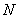is the number of scores. Mode: Mode of a set of data is the value in the set that occurs most often. Range: The range of a set of data is the difference between the highest and lowest values in the set. Variance:  The variance of a sample measures how the conservations are spread around the mean. Standard Deviation: The standard deviation is the square root of the average of the squared deviations from the mean (variance). Categorical frequency distributions: Categorical frequency distributions can be used for data that can be placed in specific categories, such as nominal- or ordinal-level data. Central Limit Theorem: As the sample size increases the sampling distribution of the sample mean approaches the normal distribution with mean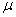and variance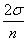. The exponential, binomial, and normal density functions: The exponential, binomial and normal density functions are used along with their respective probability distributions to approximate statistical models and values.

Rapid Study Kit for "Title":
 Flash Movie Flash Game Flash Card Core Concept Tutorial Problem Solving Drill Review Cheat Sheet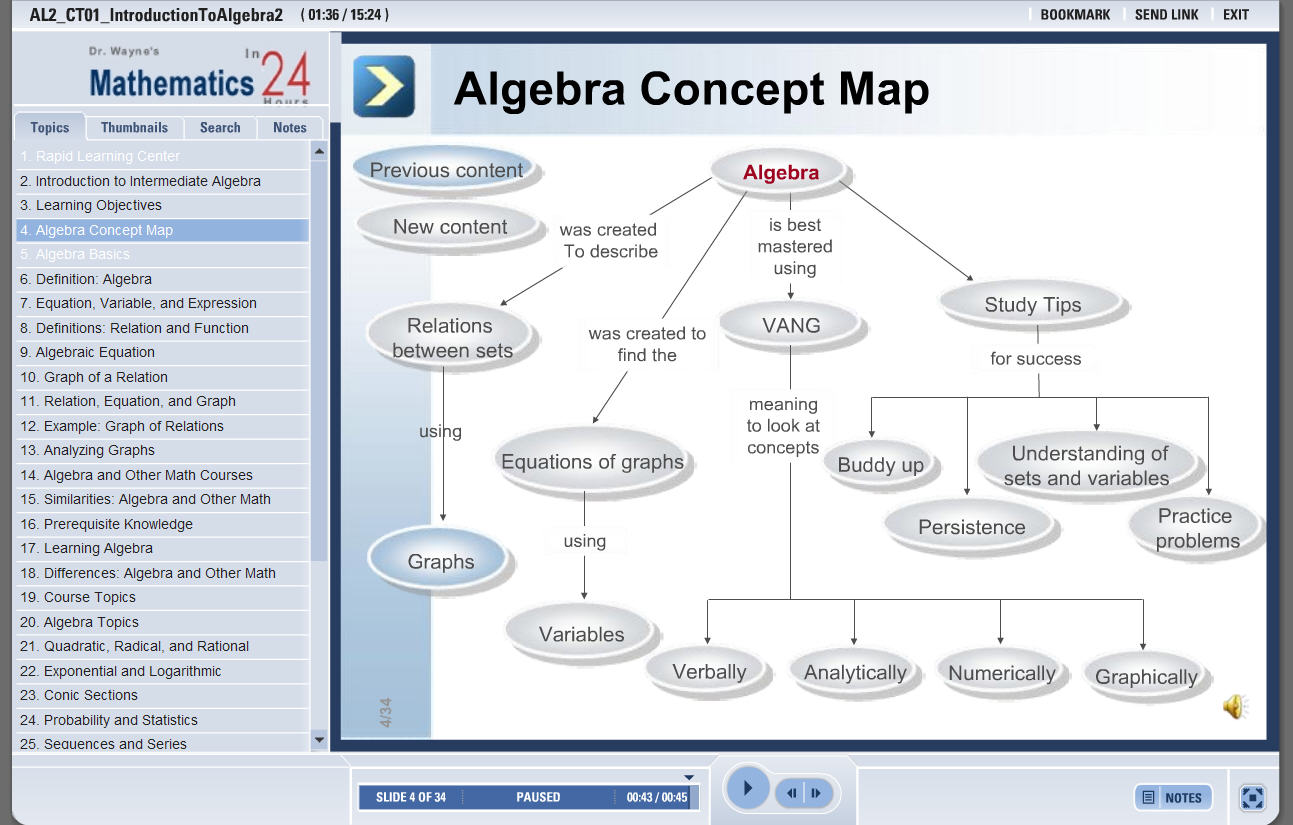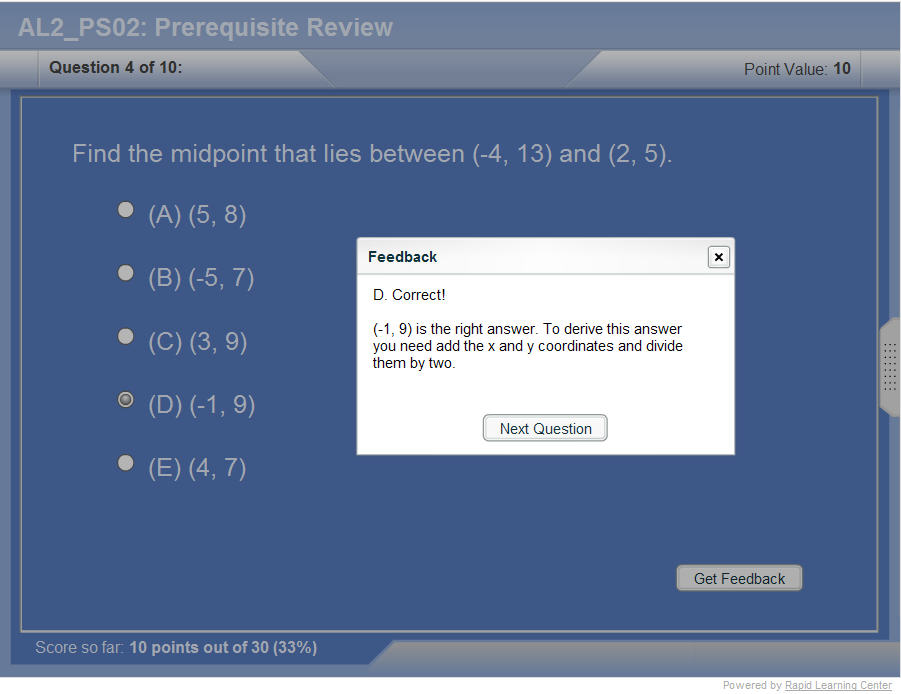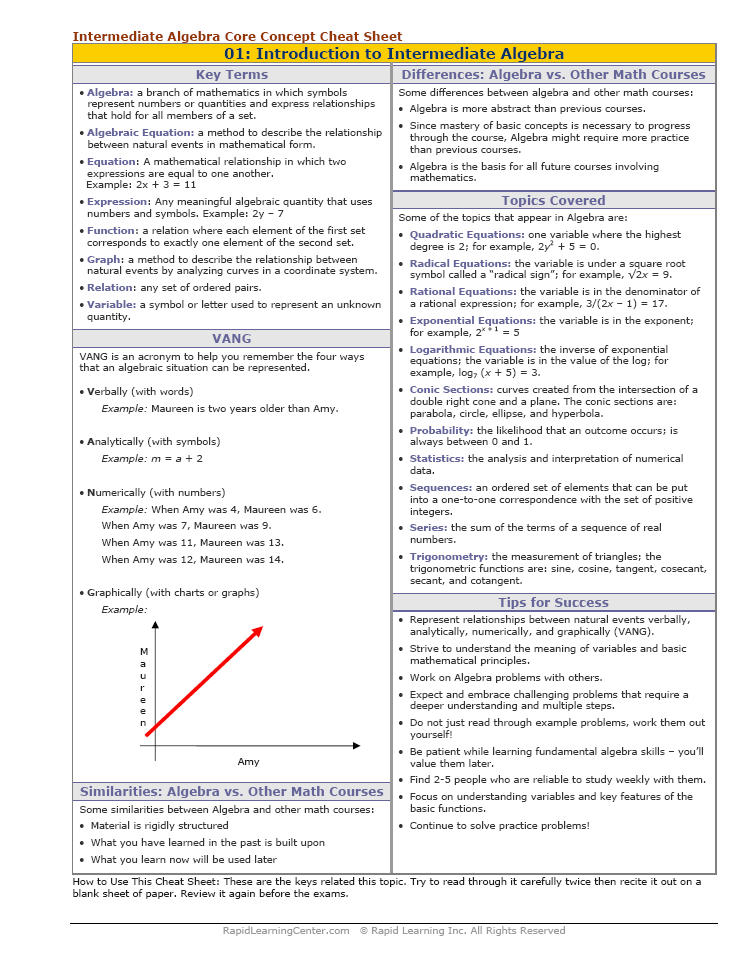"Title" Tutorial Summary : This tutorial mentions statistics and how it is applied in other mathematics courses. Statistics can be solved with problem solving methods which are mentioned in some of the examples. Histograms and bar charts in statistics are understood better with the knowledge of data and its different aspects. Graphical representation is use to represent the measures of central tendency. Statistical problems use the measures of variability, range, deviation and standard deviation. Hypothesis testing and the different types of error are presented in this tutorial. The different types of probability distributions are applied to problems using the Central Limit Theorem.

 Tutorial Features: Specific Tutorial Features: • The calculation of the mean, mode and variance are presented in some of the examples. • Examples of hypothesis testing using different alternatives. Series Features: • Concept map showing inter-connections of new concepts in this tutorial and those previously introduced. • Definition slides introduce terms as they are needed. • Visual representation of concepts • Animated examples—worked out step by step • A concise summary is given at the conclusion of the tutorial.

 "Title" Topic List: Statistics and its concepts Problem solving methods in statistics Measures of central tendency Mean Mode Midrange Range Variance Standard Deviation Random Variable Frequency Distribution and its graphStem-and-Leaf PlotProbability Distribution GraphTesting HypothesisAlternative and Null HypothesisCentral Limit Theorem

See all 24 lessons in Intermediate Algebra, including concept tutorials, problem drills and cheat sheets:
Teach Yourself Intermediate Algebra Visually in 24 Hours